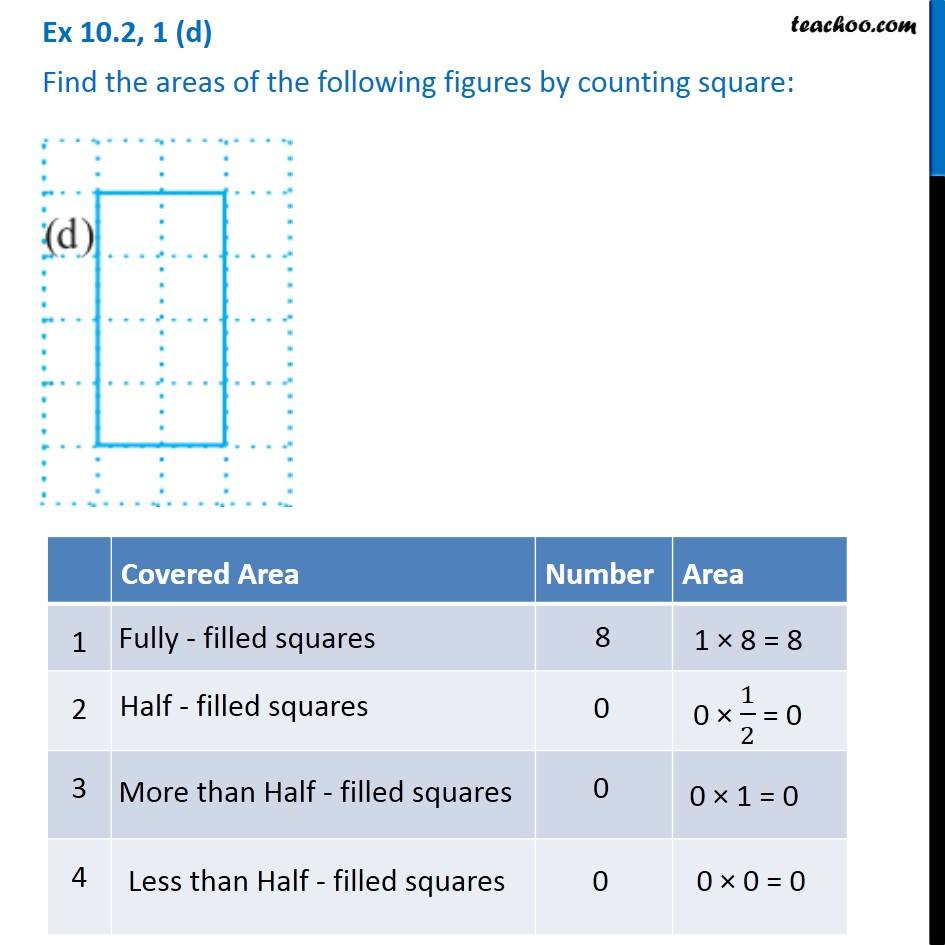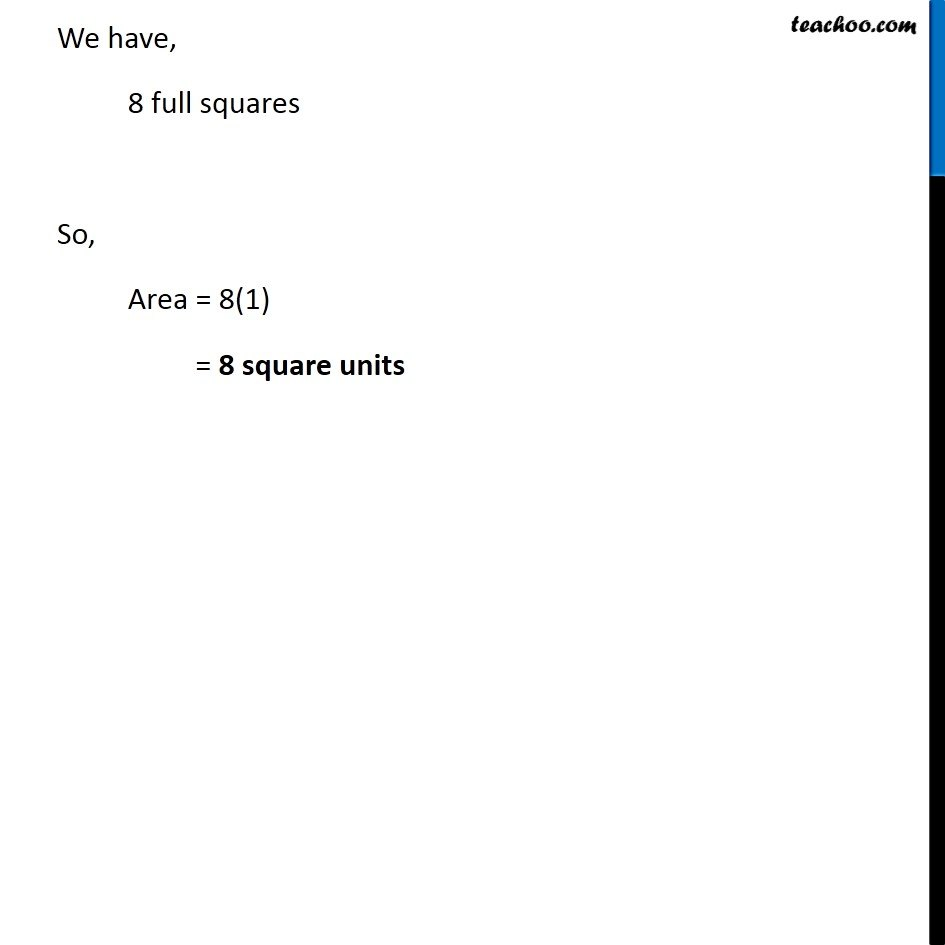Subscribe to our Youtube Channel - https://you.tube/teachoo

1. Chapter 10 Class 6 Mensuration
2. Concept wise
3. Finding area by counting squares

Transcript

Ex 10.2, 1 (d) Find the areas of the following figures by counting square: Covered Area Number Area 1 Fully filled squares 8 1 × 8 = 8 2 Half filled squares 0 0 × 1/2 = 0 3 More than Half filled squares 0 0 × 1 = 0 4 Less than half filled squares 0 0 × 0 = 0 We have, 8 full squares So, Area = 8(1) = 8 square units

Finding area by counting squares